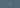# Monitor ethOS Distro with Elastic Search - 3

December 27th, 2017 - ITSoftwareTutorial(1 min)Once we’ve set up the data sending from out ethOS machine, we can check that ElasticSearch collects data. With `GET /ethos/_count`, we get something like:

``````{
"count": 5319,
"_shards": {
"total": 5,
"successful": 5,
"skipped": 0,
"failed": 0
}
}``````

If the count is greather than `0`, we can start analysing the data.

## Metrics

I am currently measuring only machine. Therefore, I’ve set up some simple measurements:

1. Hash rate
2. Temperature
3. Network
4. Fan speed

### Hash rate

I am measuring the average sum of all GPUs’ hash rates and the trend:

I’ve built this with Timelion and the code is:

``````.es(metric=avg:status.sum_hashes).points().label(),

.es(_exists_:status.sum_hashes).if(eq,0,null,
.es(metric="sum:status.sum_hashes")
).fit(average).label("Hashes"),

.es(metric=avg:status.sum_hashes).trend(linear).label(Trend)``````

As you can see, there are three components:

1. The first expression produces the (cyan) points in the picture.
2. The second expression draws the (red) lines. In order to avoid jumping to 0 for empty datasets, I’m filtering out the null values.
3. The last component produces the (yellow) trend line.

### The tempratures

The second most important element is the temperature. To put it simple: the lower the temperatures, the better for the card.

This was also created with timelion:

``````.es(_exists_:status.a_temp).if(eq, 0, null,
.es(metric="min:status.a_temp")
).fit(average).label("min"),

.es(_exists_:status.a_temp).if(eq, 0, null,
.es(metric="avg:status.a_temp")
).fit(average).label("avg"),

.es(_exists_:status.a_temp).if(eq, 0, null,
.es(metric="max:status.a_temp")
).fit(average).label("max")``````

For this, I’m measuring also three components:

1. the minimum
2. The average
3. The maximum

At the time of measurement, the rig is in an open space without dedicated air flow and, as you can see, the deperature varies. I am expecting that once the airflow is in place, the temperatures’ gap will drop and they will be lower.

### The network

Another measurement I am monitoring is the network traffic. This is not much, but I’ll be able to see when the network is down:

This is yet another Timelion graph:

``````.es(_exists_:status.val_rx_kbps).if(eq,0,null,
.es(metric="sum:status.val_rx_kbps")
).fit(average).label("Rx"),

.es(_exists_:status.val_tx_kbps).if(eq,0,null,
.es(metric="sum:status.val_tx_kbps")
).fit(average).label("Tx").yaxis(label="kbps")``````

It shows the sent and received data.

### The fan speed

I’ve also decided to implement the fan speed as agauge, simply because my setting sets the fan speed at 50%:

The metric is `Average status.fan_percents`.

HTH,

Share on

A little experiment: